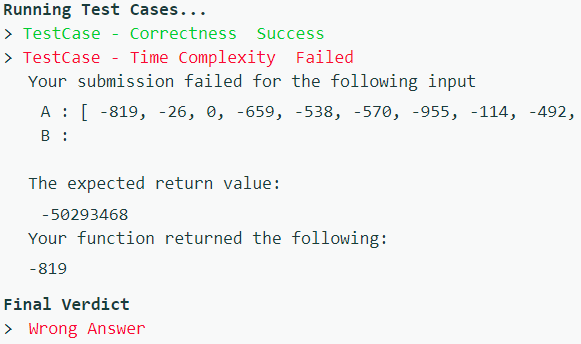# Can't figure out the wrong test-case

Code is not working in some large test-case whose B is not visible in the failed test case shown in the output section of the website. Time complexity: O(B), space complexity: O(1). Checked many test cases, works fine.

``````int Solution::solve(vector<int> &A, int B) {

vector <int> A2, A3;
A2 = A;
A3 = A;

int sum = 0;
for (int i = 0;i < B; i++){
sum += A3[i];
A3[i] = sum;
}

sum = 0;
for (int i = A.size() - 1;i > A.size() - 1 - B; i--){
sum += A2[i];
A2[i] = sum;
}

// for (int i = 0; i< A.size(); i++){
//     cout << A[i] << " ";
// }
// cout << endl;
// for (int i = 0; i < A2.size(); i++){
//     cout << A2[i] << " ";
// }
// cout << endl;

if (B > A.size()){
return -1;
}

sum = INT_MIN;
for (int i = 0; i < B-1; i++){
if (A3[i] + A2[A.size() - (B - (i+1))] > sum){
// cout << A[i] << " " << A2[A.size() - (B - (i+1))] << endl;
sum = A3[i] + A2[A.size() - (B - (i+1))];
// cout << ".";
}
}
if (A3[B-1] > sum){
sum = A3[B-1];
// cout << ".";
}
if (A2[A.size() - B] > sum){
sum = A2[A.size() - B];
// cout << ".";
}

return sum;
}
``````Hey did you find out what was the error? I’m also getting this error.